###### 400万+工程师在用###### 400万+工程师在用+加入圈子

1 、著名的Nyquist采样定理

Let x(t) be a band-limited signal with X(jw) = 0 for |w|> wM. Then x(t) is uniquely determined by its samples x(nT),n=1,±1,±2,...,ifws> 2wMwhere ws= 2 pi/T.

Given these samples, we can reconstruct x(t) by generating a periodic impluse train in which successive impluse have amplitudes that are successive sample values. This impluse train is then processed through an ideal lowpass filter with gain T and cutoff frequency greater than wMand less than ws-wM. The resulting output signal will exactly equal x(t).

2、 模数转换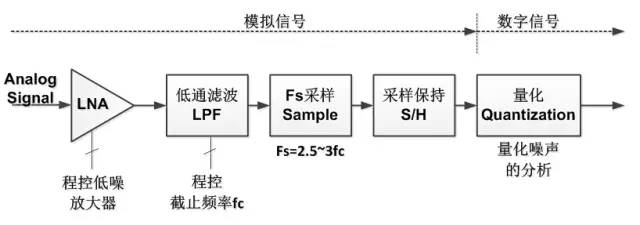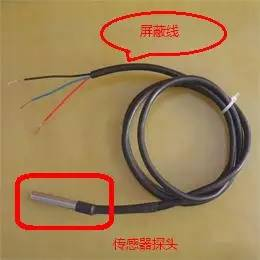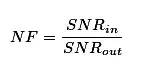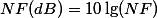NOTES: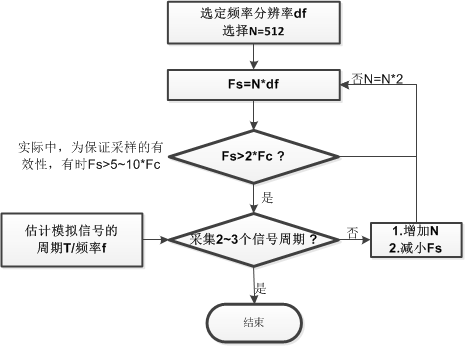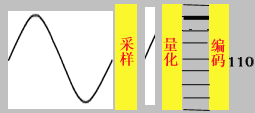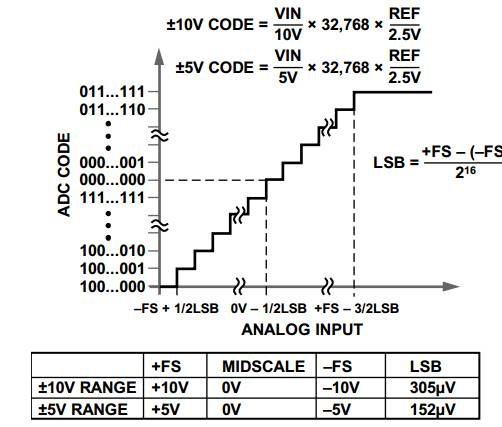±5V: (5V-(-5V)) / (2^16) = 152 uV

±10V: (10V-(-10V)) / (2^16) = 305 uV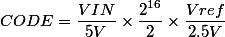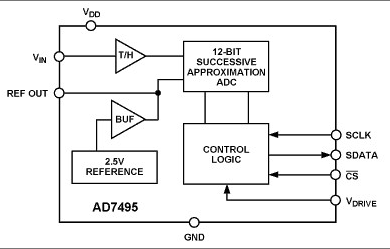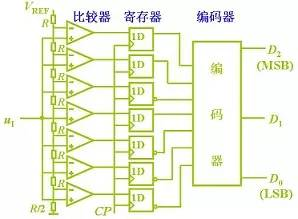SNRq= (6.02N + 4.77) dB

N=12, SNRq≈ 70dB

N=16, SNRq≈ 94dB